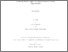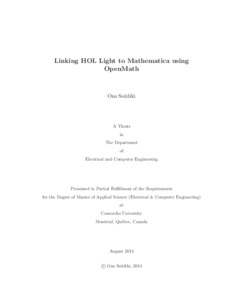Title:

# Linking HOL Light to Mathematica using OpenMath

Seddiki, Ons (2014) Linking HOL Light to Mathematica using OpenMath. Masters thesis, Concordia University.Preview Text (application/pdf) Seddiki_MSc_F2014.pdf - Accepted Version 1MB

## Abstract

One of the most important benefits of using a theorem prover system is the absolute accuracy of the obtained result. However, solving mathematical problems
often requires both deductive reasoning and algebraic computation. This issue is due to the fact that many real-life problems can be described with equations for which
we cannot find easily symbolic (or closed-form) solutions and therefore we are not able to formalize them using the theorem prover. In other cases, some applications
require well developed libraries and a deep knowledge of the theories to formalize simple expressions. A straightforward way to overcome these issues is the use of
computer algebra systems or numerical approaches which are known to be the most efficient tools in symbolic computation. However, to preserve the soundness of the
computation, the results of these systems should be formally verified. In this thesis, we present a general architecture to connect HOL Light, a higher-order logic theorem prover, to any mechanized mathematical system that supports the mathematical standard OpenMath. We implemented a prototype, called HolMatica, which links HOL Light to the computer algebra system Mathematica through OpenMath. We
describe our implementation of a HOL Light translator which converts HOL Light statements into OpenMath object and vice-versa.

Divisions: Concordia University > Gina Cody School of Engineering and Computer Science > Electrical and Computer Engineering Thesis (Masters) Seddiki, Ons Concordia University M.A. Sc. Electrical and Computer Engineering 22 August 2014 Tahar, Sofiene 978965 ONS SEDDIKI 04 Nov 2014 15:24 18 Jan 2018 17:48
All items in Spectrum are protected by copyright, with all rights reserved. The use of items is governed by Spectrum's terms of access.

Repository Staff Only: item control pageResearch related to the current document (at the CORE website)
Back to top